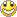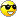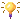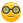Some new stuff
1. Arduino Park Control
2. Arduino USB2serial programming the bare bone
3. Arduino Projects Videos added
4. Arduino Xbee projects
5. Arduino dB Meter
6. Trying to get the IOIO going.
7. IOIO is going....bluetooth8. Attiny 2313 bluetooth bee RC car
9. Compass and temperature added
10. AVR programmer
11. USB2Serial and Ethernet POE
12. Arduino LPG Sensor
13. Program the Arduino Mini 05
14. Lipo Rider Pro in action
15. EZ Robot Builder kit arrived 10 Nov 2012
16. Arduino VA Meter!
17. 05 Nov 2012 Project Sentry Gun.
18. Attiny85 PIR Sensor 10 Dec 2012
19. 0-30VDC3A and 2-28VDC10A bench PSU's20. 19 Dec 2012 Arduino Voltmeter LCD
21. 29 Dec 2012 Arduino Tachometer&Speedometer LCD
22. 03 Jan 2013 Arduino AC Phase Control.
23. 18 Jan 2013 Arduino Distance Meter
24. 22 Jan 2013 Arduino Digital Clock and Date
25. 31 Jan 2013 Arduino Digital Clock and Date 8x2 LCD
26. Feb 2013 LCD and Backpack
27. Feb 2013 7 Segment Serial Clock Sparkfun. Distance Sensor with "newping" library
28. Feb 2013 Lelo Remote
29. 01/03/2013 Arduino Adafruit 7 Seg Digital Clock with RTC and backpack
30. Arduino Frequency Meter 26/03/2013
31. Another RC  Car controlled by Arduino 06/04/2013
32. My quadcopter project update 25 April 2013
33. My Aqua Quad Copter Flying 26 April 201334. Another monster RC truck;strong one! 11July201335. X-Frame Quad Copter 31 July 201336. Arduino GSM Sheild added.28/8/2013
37. 1.8inch TFT screen 13/09/201338. 04/11/2014 added PID Soldering Iron Control
39. Added bluetooth servo control 04/11/2014
40. ESP8266 first try.16/03/2015
41. Arduino Uno Quadcopter 19 May 2015
42. IOT WemosD1 Amp Meter26/03/2013

Arduino Frequency Counter up to 6 MHz.The code is not mine, but once again I changed the code to accommodate the LCD 16 x 2 backpack Adafruit.

/*
Frequency Counter Arduino Sketch
by: Jim Lindblom
SparkFun Electronics
License: Beerware

Input d5 via a 10K resistor to ground.

This isn't the most accurate frequency counter, but it's simple to
program and understand, and it works in a pinch. I've tested it to
be accurate to at least 96% (usually around 99.5%). It should be
able to measure frequencies up to about 6.5MHz(8MHz optimally)

The circuit: Powered at 5V (5V LCD), Arduino running at 16MHz*/
#include "Wire.h"
#include "LiquidCrystal.h"
// A4 = SDA,A5 = SCL
LiquidCrystal lcd(0);
// initialize the library with the numbers of the interface pins
int brightness;
unsigned int tovf1 = 0;
unsigned long frequency = 0;
unsigned long temp = 0;

// Timer 1 is our counter
// 16-bit counter overflows after 65536 counts
// tovfl will keep track of how many times we overflow
ISR(TIMER1_OVF_vect)
{
tovf1++;
}

void setup() {
pinMode(5, INPUT);  // This is the frequency input
// Timer 1 will be setup as a counter
// Maximum frequency is Fclk_io/2
// (recommended to be < Fclk_io/2.5)
// Fclk_io is 16MHz
TCCR1A = 0;
// External clock source on D5, trigger on rising edge:
TCCR1B = (1<   // Enable overflow interrupt
// Will jump into ISR(TIMER1_OVF_vect) when overflowed:
TIMSK1 = (1<
// set up the LCD's number of rows and columns:
lcd.begin(16, 2);

// Print a splash screen to the LCD.
lcd.print("FrequencyCounter");
lcd.setCursor(0, 1);
lcd.print("      v1.0      ");
delay(2000);
}

void loop() {
// Delay a second. While we're delaying Counter 1 is still
// reading the input on D5, and also keeping track of how
// many times it's overflowed
delay(1000);

lcd.clear();

frequency = (TCNT1H<<8)|TCNT1L;
frequency = (TCNT1H<<8)|TCNT1L;

// Correct weird counter bug
// A small range of frequencies (~30k-50k) are getting
// 42949 appended to the front of them
// Will look into this but this works for now
if (frequency > 40000000)
frequency -= 4294900000;

// 65536 (2^16) is maximum of counter
// We'll multiply that by how many times we overflowed
temp = 65536*(unsigned long)tovf1;

// Add the overflow value to frequency
frequency += temp;

// Print the proper amount of spaces to make it look pretty
lcd.setCursor(0,1);
if (frequency < 100)
lcd.print("      ");
else if (frequency < 10000)
lcd.print("     ");
else if (frequency < 1000000)
lcd.print("    ");
else
lcd.print("   ");
lcd.print(frequency);
lcd.print(" Hz");

// Reset all counter variables and start over
TCNT1H = 0;
TCNT1L = 0;
tovf1 = 0;
}

And the code for 8x2 LCD on own build arduino sheild:/*
Input via a 10K resistor to ground on Pin 2.

*/

#include <LiquidCrystal.h>
// initialize the library with the numbers of the interface pins
LiquidCrystal lcd(8,9,4,5,6,7);
unsigned int tovf1 = 0;
unsigned long frequency = 0;
unsigned long temp = 0;
// Timer 1 is our counter
// 16-bit counter overflows after 65536 counts
// tovfl will keep track of how many times we overflow
ISR(TIMER1_OVF_vect)
{
tovf1++;
}
void setup() {
pinMode(2, INPUT);  // This is the frequency input
// Timer 1 will be setup as a counter
// Maximum frequency is Fclk_io/2
// (recommended to be < Fclk_io/2.5)
// Fclk_io is 16MHz
TCCR1A = 0;
// External clock source on D5, trigger on rising edge:
TCCR1B = (1<   // Enable overflow interrupt
// Will jump into ISR(TIMER1_OVF_vect) when overflowed:
TIMSK1 = (1<   // set up the LCD's number of rows and columns:
lcd.begin(8, 2);
// Print a splash screen to the LCD.
lcd.print("Freq = ");
delay(2000);
}
void loop() {
// Delay a second. While we're delaying Counter 1 is still
// reading the input on D2, and also keeping track of how
// many times it's overflowed
delay(1000);
lcd.setCursor(0,0);
lcd.print("Freq = ");
frequency = (TCNT1H<<8)|TCNT1L;
frequency = (TCNT1H<<8)|TCNT1L;
// Correct weird counter bug
// A small range of frequencies (~30k-50k) are getting
// 42949 appended to the front of them
// Will look into this but this works for now
if (frequency > 40000000)
frequency -= 4294900000;
// 65536 (2^16) is maximum of counter
// We'll multiply that by how many times we overflowed
temp = 65536*(unsigned long)tovf1;
// Add the overflow value to frequency
frequency += temp;
// Print the proper amount of spaces to make it look pretty
lcd.setCursor(0,1);
if (frequency < 100)
lcd.print("     ");
else if (frequency < 10000)
lcd.print("     ");
else if (frequency < 1000000)
lcd.print("    ");
else
lcd.print("   ");
lcd.print(frequency);
lcd.print("Hz");
// Reset all counter variables and start over
TCNT1H = 0;
TCNT1L = 0;
tovf1 = 0;
}

 Back Back to top